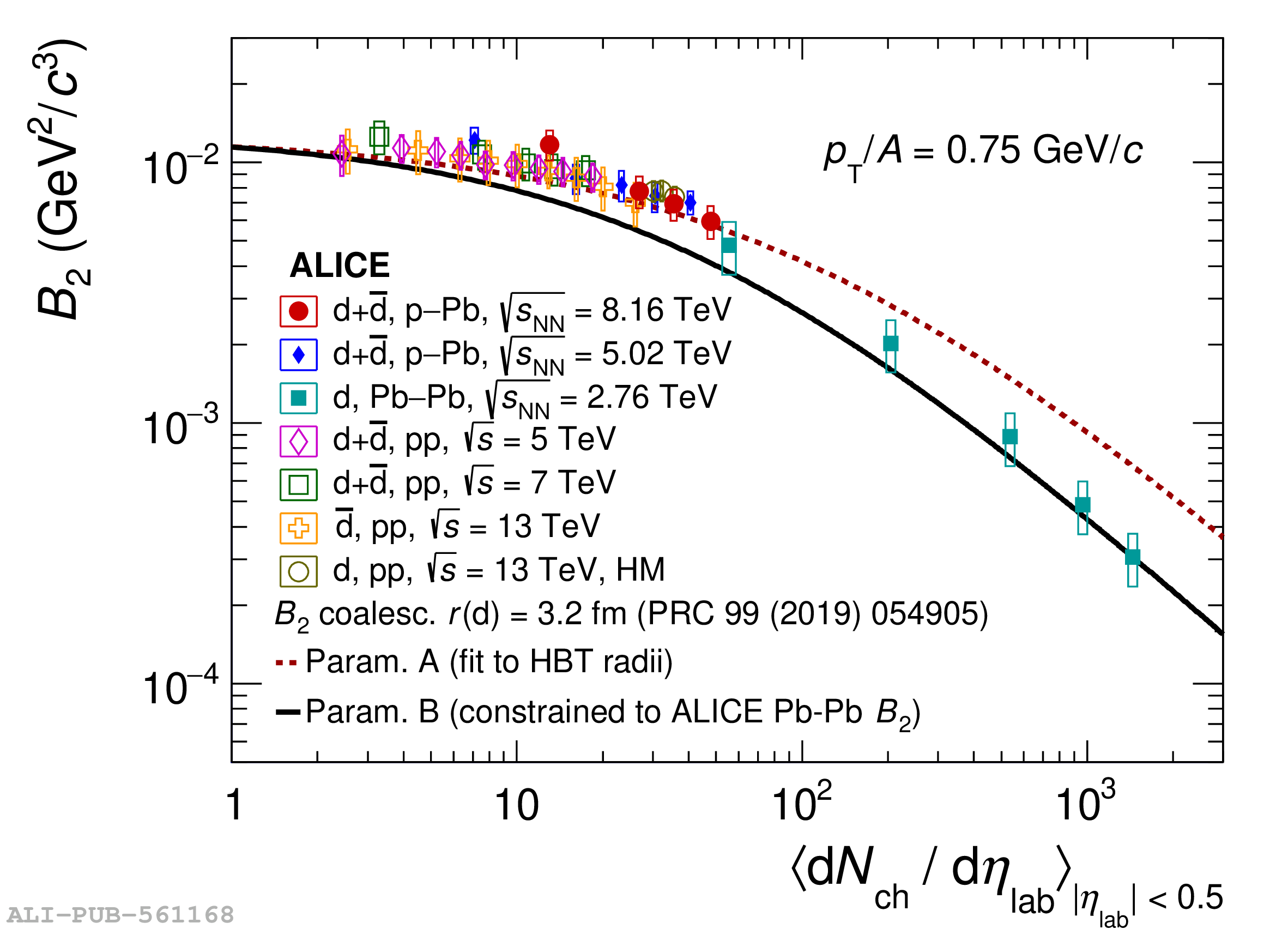# Figure 3

 $B_2$ as a function of the mean charged-particle multiplicity density for a fixed value of \mbox{$p_{\mathrm T}$/$A$ = 0.75 GeV/$c$}. The experimental results are compared to the coalescence calculations from Ref.  using two different parameterizations for the system size as a function of the mean charged-particle multiplicity density.